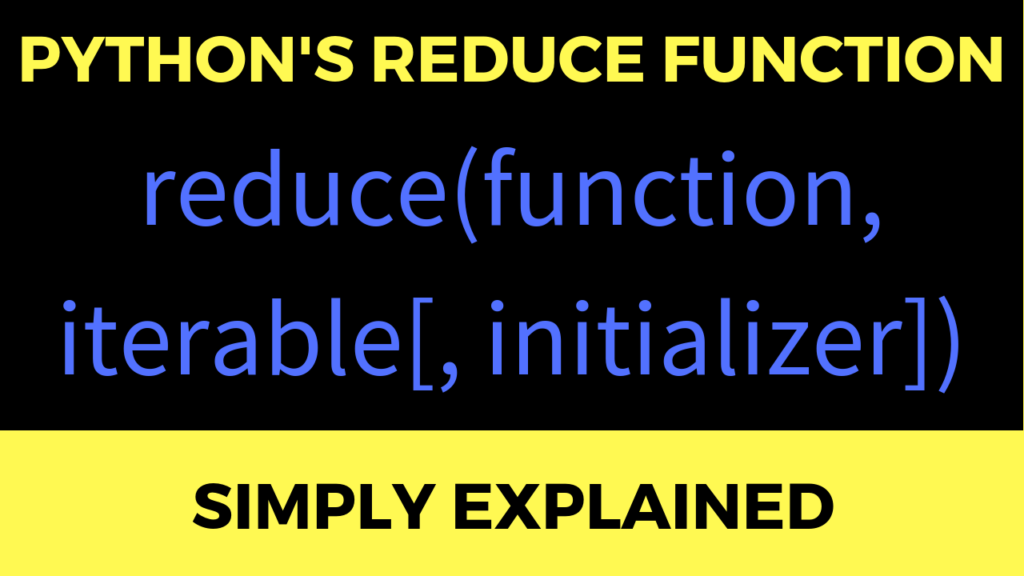# The Reduce Function in Python 3: Simply Explained

The reduce function in Python reduces a sequence of elements to a single element by repeatedly applying a specified function that takes two sequence elements and merges them to a single element.

By repeating this behavior, ultimately, only a single value will remain — the sequence is reduced to a single element.

Here is an example (one-liner code puzzle): What is the output of this Python one-liner?

```from functools import reduce
print(reduce(lambda x, y: x * y, range(1, 6))) ```

I gave this puzzle to 3,453 Python coders in my Finxter community. Six of them replied with the correct solution: The one-liner calculates the factorial function “5!”.

Thus, the result is

(((((1 * 2) * 3) * 4) * 5) = 120

As you can guess from the formatting, the reduce function iteratively combines two values from an iterable as specified in the first functional argument. In our case, the functional argument is an anonymous (lambda) function that takes two values, multiplies them, and returns the result.

Let’s keep exploring the reduce function further: what is the output of the following one-liner code puzzle? Let’s explore yesterday’s one-liner puzzle:

```from functools import reduce
print(reduce(lambda x, y: x + [[z for z in range(y)]], [1, 2, 3, 4], [ ])) ```

Yes, the solution is:

Solution: [, [0, 1], [0, 1, 2], [0, 1, 2, 3]]

But how does this Python one-liner work?

The reduce function iteratively combines two values from an iterable as specified in the first functional argument. In our case, the functional argument is an anonymous (lambda) function that takes two values x and y, combines them, and returns the result.

In the previous one-liner code puzzle, we combined the two (integer) values by multiplying them (and the result was the factorial function).

But in this puzzle, our two values x and y have a different data type. The third argument of the reduce function specifies the initial value of x. You can see that the initial value of x is an empty list.

However, value y still takes on each integer value of the list to be reduced (i.e., the second argument of the reduce function). So, we have y=1, y=2, y=3, and y=4.

Now, we repeatedly merge value y into the list x. Basically, we create a new list using list comprehension. The new list consists of all integer values up to y (exclusive).

This new list is then added to the old list (which was initially empty and is growing steadily).

Here is the exact procedure for each integer y in the list to be reduced:
(the right-hand side shows you how the new list is merged into the old list in each reduce step.)

y=1: [] + [] = []
y=2: [] + [[0,1]] = [, [0,1]]
y=3: [, [0,1]] + [[0,1,2]] = [, [0,1], [0,1,2]]
y=4: [, [0,1], [0,1,2]] + [[0,1,2,3]] = [, [0,1], [0,1,2], [0,1,2,3]]

I will hammer the reduce function into your brain (if it’s the last thing I do). So let’s solve another reduce puzzle: what is the output of this one-liner?

```from functools import reduce
print(reduce(lambda x,y: x|{y}, [1, 1, 1, 1, 2, 3, 4], {0})) ```

Correct, it’s {0,1,2,3,4}.

If you don’t have a lot of time to study Python for hours and hours, join my free “Coffee Break Python” email course. I will send you a daily Python lesson, cheat sheets, and we are doing a weekly code contest. It’s fun (and 100% free)!

*** Yes, show me how I can participate! ***